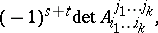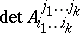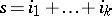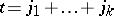# Cofactor

(diff) ← Older revision | Latest revision (diff) | Newer revision → (diff)
Jump to: navigation, search

for a minorThe numberwhereis a minor of order, with rowsand columns, of some square matrixof order;is the determinant of the matrix of orderobtained fromby deletion of the rows and columns of;,. Laplace's theorem is valid: If anyrows are fixed in a determinant of order, then the sum of the products of the minors of the-th order corresponding to the fixed rows by their cofactor is equal to the value of this determinant.

#### Comments

This Laplace theorem is often referred to as Laplace's development of a determinant.

How to Cite This Entry:
Cofactor. Encyclopedia of Mathematics. URL: http://encyclopediaofmath.org/index.php?title=Cofactor&oldid=39509
This article was adapted from an original article by V.N. Remeslennikov (originator), which appeared in Encyclopedia of Mathematics - ISBN 1402006098. See original article